# Elastic Net SearcheR Examples

### ensr package version 0.1.0

#### 2019-01-14

The primary purpose of the ensr package is to provide methods for simultaneously searching for preferable values of $$\lambda$$ and $$\alpha$$ in elastic net regression. ensr is wrapped around the r Rpkg("glmnet") package This vignette starts with a summary of elastic net regression and its use and limitations. Examples of data set preparation follow and the vignette concludes with elastic net regression results.

library(ensr)
##
## Attaching package: 'glmnet'
## The following object is masked from 'package:qwraps2':
##
##     auc
library(data.table)
library(ggplot2)
## Registered S3 methods overwritten by 'ggplot2':
##   method         from
##   [.quosures     rlang
##   c.quosures     rlang
##   print.quosures rlang
library(ggforce)
library(doMC)
registerDoMC(cores = max(c(detectCores() - 2L, 1L)))
options(datatable.print.topn  = 3L,
datatable.print.nrows = 3L)

# 1 Elastic Net Regression

Elastic Net Regression (Friedman, Hastie, and Tibshirani 2010) is a penalized linear modeling approach that is a mixture of ridge regression (Hoerl and Kennard 1970), and least absolute shrinkage and selection operator (LASSO) regression (Tibshirani 1996). Ridge regression reduces the impact of collinearity on model parameters and LASSO reduces the dimensionality of the support by shrinking some of the regression coefficients to zero. Elastic net does both of these by solving the following equation (for Gaussian responses): $\min_{\beta_0, \beta \in \mathbb{R}^{p+1}} \frac{1}{2N} \sum_{i = 1}^{N} \left( y_i - \beta_0 - x_i^T \beta \right)^2 + \lambda \left[ \left(1 - \alpha \right) \frac{\left \lVert \beta \right \rVert_{2}^{2}}{2} + \alpha \left \lVert \beta \right \rVert_{1} \right],$ where $$\lambda \geq 0$$ is the complexity parameter and $$0 \leq \alpha \leq 1$$ is the compromise between ridge $$\left(\alpha = 0\right)$$ and LASSO $$\left( \alpha = 1 \right).$$

Ridge regression does not often shrink coefficients to zero and contribute to the parsimony of models. One potential benefit of elastic net regression is that, like the LASSO, it can be used to perform variable selection by shrinking coefficients to zero. Compared to LASSO, one potential benefit of elastic net regression is that it will reproducibly return the same set of non-zero coefficients when some predictors are highly correlated. LASSO, $$\alpha = 1,$$ may return different sets of non-zero coefficients when highly correlated predictors are in the model.

Compared to other machine learning approaches, a potential benefit of elastic net regression is that the $$\beta$$ vector is easily interpretable and can be implemented in almost any downstream computational pipeline. More flexible machine learning models such as gradient boosting machines may be able to fit data more accurately, but they are extremely difficult to export to other tools.

The cv.glmnet call from the glmnet package is widely used to fit elastic net regression models. However, the current implementation of cv.glmnet requires that the value(s) of $$\alpha$$ be specified by the user (see “Details” in help("cv.glmnet")). We designed the ensr package to fill this gap by simultaneously searching for a preferable set of $$\lambda$$ and $$\alpha$$ values. ensr also provides additional plotting methods to facilitate visual identification of the best choice for a given project.

# 2 Data Sets

Two data sets are provided in the ensr package for use in examples.

1. tbi is a synthetic data set with which traumatic brain injury can be classified into three different types using a set of predictors.

2. landfill is a synthetic data set similar to those generated by computer models of water percolating through landfill.

vignette("ensr-datasets", package = "ensr")
data(tbi, package = "ensr")
data(landfill, package = "ensr")

# 3 Searching for $$\lambda$$ and $$\alpha$$

The optimal pair of $$\lambda$$ and $$\alpha$$ is likely project-specific. Some defaults are provided but the user is encouraged to carefully consider, for example, the optimal balance between parsimony and model error for their project. For example, model error that is lower by 0.1% at the expense of 3 additional parameters may or may not be desirable.

## 3.1 Univariate Response

A call to ensr produces a search for a combination of $$\lambda$$ and $$\alpha$$ that result in the lowest cross validation error. The arguments to ensr are the same as those made to cv.glmnet with the addition of alphas, a sequence of $$\alpha$$ values to use. Please note that ensr will add length(alphas) - 1 additional values, the midpoints between the given set, in the construction of a $$\lambda$$$$\alpha$$ grid to search. For the initial example we will fit an elastic net for modeling the evaporation in the landfill data constructed above.

set.seed(42)
y_matrix <- as.matrix(landfill$evap) x_matrix <- as.matrix(landfill[, topsoil_porosity:weather_temp]) ensr_obj <- ensr(y = y_matrix, x = x_matrix, standardize = FALSE) ensr_obj ## A ensr object with 19 cv.glmnet objects. ## List of 19 ## - attr(*, "class")= chr "ensr" ensr_obj is a ensr object, which is a list of cv.glmnet objects. The length of the list is determined by the length of the $$\alphas$$ argument. The default for alphas is seq(0, 1, length = 10). The summary method for ensr objects returns a data.table with values of $$\lambda$$, $$\alpha$$, the mean cross-validation error cvm, and the number of non-zero coefficients. The l_index is the list index of the ensr object associated with the noted $$\alpha$$ value. ensr_obj_summary <- summary(object = ensr_obj) ensr_obj_summary ## l_index lambda cvm nzero alpha ## 1: 1 4.024266e+03 0.9074317189 35 0 ## 2: 1 3.341016e+03 0.9073969721 35 0 ## 3: 1 2.773770e+03 0.9073551226 35 0 ## --- ## 13749: 19 4.427853e-05 0.0009092816 32 1 ## 13750: 19 4.421884e-05 0.0009092857 32 1 ## 13751: 19 4.416627e-05 0.0009092893 32 1 The preferable model may be the one with the minimum cross-validation error. ensr_obj_summary[cvm == min(cvm)] ## l_index lambda cvm nzero alpha ## 1: 13 0.0007578241 0.0008922982 18 0.6666667 A quick way to get the preferable model is to call the preferable method. str(preferable(ensr_obj), max.level = 1L) ## List of 13 ##$ a0       : Named num [1:819] -0.00196 -0.001236 -0.000305 0.000437 0.002263 ...
##   ..- attr(*, "names")= chr [1:819] "s0" "s1" "s2" "s3" ...
##  $beta :Formal class 'dgCMatrix' [package "Matrix"] with 6 slots ##$ df       : int [1:819] 1 1 1 1 1 1 1 1 1 1 ...
##  $dim : int [1:2] 35 819 ##$ lambda   : num [1:819] 0.604 0.594 0.582 0.573 0.551 ...
##  $dev.ratio: num [1:819] 1.30e-15 1.03e-02 2.33e-02 3.35e-02 5.84e-02 ... ##$ nulldev  : num 878
##  $npasses : int 1913 ##$ jerr     : int 0
##  $offset : logi FALSE ##$ call     : language glmnet(x = x_matrix, y = y_matrix, lambda = c(7.03251863470795e-05,  8.47069359244159e-05, 0.000102029804205433, | __truncated__ ...
##  $nobs : int 974 ##$ cv_row   :Classes 'ensr_summary', 'data.table' and 'data.frame':  1 obs. of  5 variables:
##   ..- attr(*, ".internal.selfref")=<externalptr>
##   ..- attr(*, "call")= language preferable.ensr(object = ensr_obj)
##  - attr(*, "class")= chr [1:3] "ensr_pref" "elnet" "glmnet"

preferable returns a elnet glmnet object with one additional list element, the ensr_summary used to select this preferable model.

Because the output of preferable inherits the same class as an object returned from a call to glmnet::glmnet the same methods can be used. Plotting methods are one example:

par(mfrow = c(1, 3))
plot(preferable(ensr_obj), xvar = "norm")
plot(preferable(ensr_obj), xvar = "lambda")
plot(preferable(ensr_obj), xvar = "dev")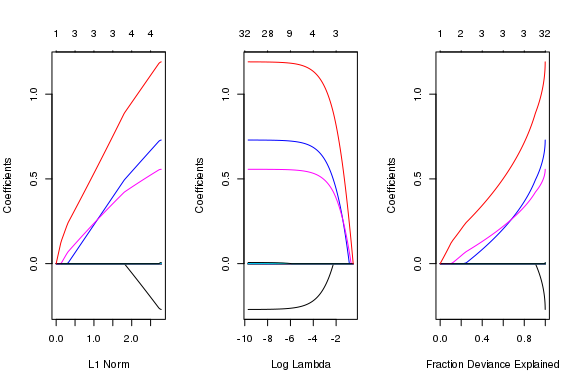Another graphical way to look at the results of the ensr is to use the ensr-provided plotting method. In the plot below, each of the $$\lambda$$ (y-axis, $$\log_{10}$$ scale) and $$\alpha$$ (x-axis) values considered in the ensr_obj are plotted. The coloring is denoted as log10(z) where z = (cvm - min(cvm)) / sd(cvm). The color scale is set to have low values (values near the minimum mean cross validation error) be dark green. Values moving further from the minimum are lighter green, then white, then purple. A red cross identifies the minimum mean cross-validation error.

plot(ensr_obj)
## Warning: Removed 1 rows containing non-finite values (stat_contour).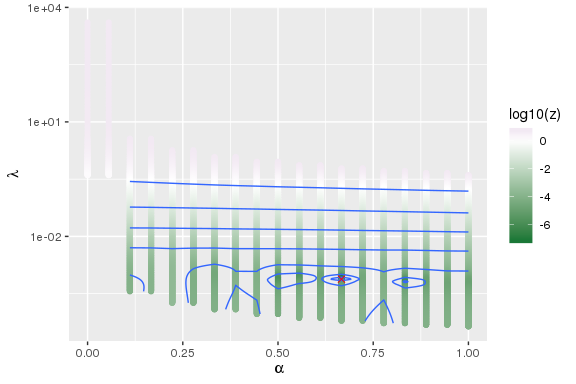The ensr plot method produces a ggplot object and thus can be customized. In this example, we add the black-and-white theme and use ggforce::facet_zoom to zoom in on a section of the graphic:

plot(ensr_obj) +
theme_minimal() +
facet_zoom(x = 0.50 < alpha & alpha < 0.90, y = 5e-4 < lambda & lambda < 1.5e-3)
## Warning: Removed 3 rows containing non-finite values (stat_contour).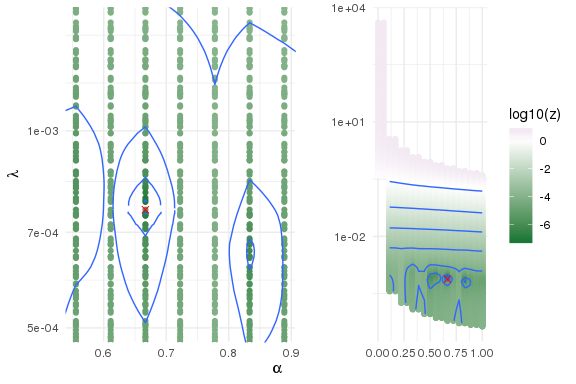In this figure we see the minimum mean cross validation error occurs within the models with $$\alpha$$ = 0.6666667.

summary(ensr_obj)[cvm == min(cvm)]
##    l_index       lambda          cvm nzero     alpha
## 1:      13 0.0007578241 0.0008922982    18 0.6666667

Inspection of the plot suggests there is another minimum worth considering, for $$\alpha$$ = 0.8333333.

summary(ensr_obj)[, .SD[cvm == min(cvm)], by = alpha][l_index %in% c(13, 16)]
##        alpha l_index       lambda          cvm nzero
## 1: 0.6666667      13 0.0007578241 0.0008922982    18
## 2: 0.8333333      16 0.0006558550 0.0008924258    17

The difference in the mean cross validation error between these two results is very small and may not be meaningful. However, the number of non-zero (nzero) coefficients is quite different. With a very small increase in the mean cross validation error, one more variable has its regression coefficient shrunk to zero. If parsimony is your primary objective, the second model might be preferable.

We can also look at the mean cross validation errors by nzero.

summary(ensr_obj)[, .SD[cvm == min(cvm)], by = nzero]
##     nzero l_index      lambda          cvm     alpha
##  1:    35       1 0.402881802 0.2579894975 0.0000000
##  2:     0      18 0.426160736 0.8943092345 0.9444444
##  3:     1      19 0.337040017 0.7560775461 1.0000000
## ---
## 32:    30      10 0.000280764 0.0008965387 0.5000000
## 33:    31      10 0.000185691 0.0008985694 0.5000000
## 34:    32      10 0.000104653 0.0009006117 0.5000000

The plot method for ensr objects has a type argument. The default is type = 1 as plotted above. type = 2 plots:

plot(ensr_obj, type = 2)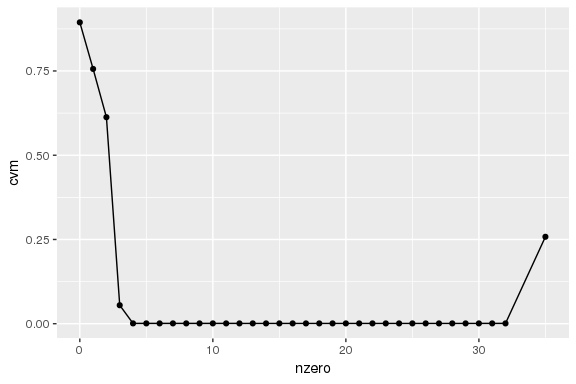Some customization to the plot:

plot(ensr_obj, type = 2) +
theme_bw() +
aes(x = nzero, y = cvm) +
geom_point() +
geom_line() +
facet_zoom(xy = cvm < 0.05)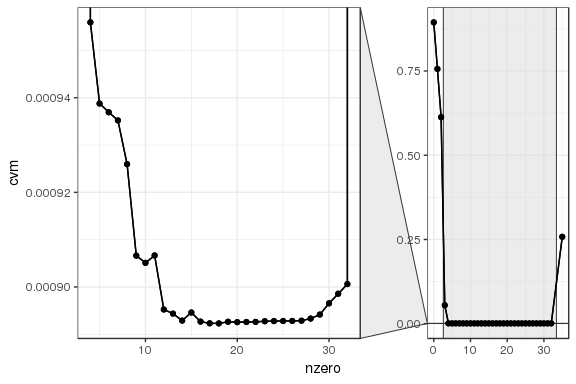Based on the figure above, if the objective is lowest cross validation error and parsimony, the model with 14 non-zero coefficients may be the preferable model. The additional non-zero coefficient in the model with 15 or more non-zero coefficients does not meaningfully reduce the mean cross validation error. Further examination shows that the model with only four non-zero coefficients might also be a reasonable choice.

summary(ensr_obj)[nzero %in% c(4, 14)] [, .SD[cvm == min(cvm)], by = nzero]
##    nzero l_index       lambda          cvm     alpha
## 1:     4      19 0.0023788208 0.0009559391 1.0000000
## 2:    14      16 0.0007415985 0.0008928932 0.8333333

A quick side note: you can get both types of plots in one call:

plot(ensr_obj, type = c(1, 2))
## Warning: Removed 1 rows containing non-finite values (stat_contour).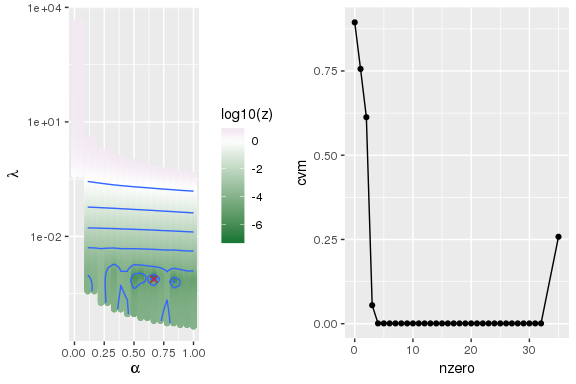To obtain the coefficients from the above models:

landfill_evap_coef4  <- coef(ensr_obj[], s = 0.0023788208)
landfill_evap_coef14 <- coef(ensr_obj[], s = 0.0007415985)

The table below shows the variables for each model in descending order of the absolute value of the regression coefficients. Because the predictors were standardized, the relative magnitude of the coefficients can serve as a sensitivity/influence/importance metric.

qwraps2::qable(
data.table(
variable = c(rownames(landfill_evap_coef4),
rownames(landfill_evap_coef14)),
value    = c(as.vector(matrix(landfill_evap_coef4)),
as.vector(matrix(landfill_evap_coef14))),
nzero    = c(rep(4, 36), rep(14, 36))
)[
abs(value) >= 1e-10 & variable != "(Intercept)"
][
order(nzero, -abs(value))
][
,
nzero := NULL
]
,
rgroup = c("nzero = 4" = 4, "nzero = 14"= 14),
rnames = c(1:4, 1:14))
variable value
nzero = 4
1 weather_temp 1.183977e+00
2 wind 7.230358e-01
4 rh -2.613265e-01
nzero = 14
1 weather_temp 1.188508e+00
2 wind 7.276644e-01
4 rh -2.680920e-01
5 weather_precip 4.449118e-03
6 topsoil_ks -1.976958e-03
7 wlt_thetar 1.794380e-03
8 cn 1.299218e-03
9 liner_pinholes 1.249752e-03
10 clay_porosity -9.975358e-04
11 topsoil_n 7.837412e-04
12 ult_thetar -5.023029e-04
13 lai 1.783396e-04
14 rmw -9.395882e-05

The variables most important for modeling evaporation are weather_temp (average temperature over the last 100 years), wind (average wind speed), weather_solrad (average solar radiation), and rh (relative humidity). The fifth and higher coefficients are considerably smaller and less important than the first four.

## 3.2 Cross Validation Issues

Cross-validation results may be dependent on the foldid randomly assigned to each record. This is because some records may always be considered together in either the training or validation data sets. For example, three randomly generated vectors for 10-fold cross-validation are generated below and the results from calls to ensr are shown below.

foldid1 <- sample(seq(10), size = nrow(x_matrix), replace = TRUE)
foldid2 <- sample(seq(10), size = nrow(x_matrix), replace = TRUE)
foldid3 <- sample(seq(10), size = nrow(x_matrix), replace = TRUE)

ensr_obj_1 <- ensr(y = y_matrix, x = x_matrix, standardize = FALSE, foldid = foldid1)
ensr_obj_2 <- ensr(y = y_matrix, x = x_matrix, standardize = FALSE, foldid = foldid2)
ensr_obj_3 <- ensr(y = y_matrix, x = x_matrix, standardize = FALSE, foldid = foldid3)

summary(ensr_obj_1)[cvm == min(cvm)]
##    l_index      lambda          cvm nzero alpha
## 1:      19 0.000655855 0.0008922834    14     1
summary(ensr_obj_2)[cvm == min(cvm)]
##    l_index       lambda          cvm nzero alpha
## 1:      19 0.0006393154 0.0009048849    14     1
summary(ensr_obj_3)[cvm == min(cvm)]
##    l_index       lambda          cvm nzero alpha
## 1:      19 0.0004662574 0.0009024661    18     1

There are small differences in the cross validation errors and in the $$\lambda$$ values. There is a large difference, however, in the $$\alpha$$ values. It is notable that the differences in the regression coefficients is minor. In this case, the number of non-zero coefficients does not change and the coefficient magnitudes are similar.

cbind(coef(ensr_obj_1), coef(ensr_obj_2), coef(ensr_obj_3))
## 36 x 3 sparse Matrix of class "dgCMatrix"
##                              1             1             1
## (Intercept)       6.114954e-02  6.114603e-02  6.096917e-02
## topsoil_porosity  .             .             .
## topsoil_alpha     .             .             .
## topsoil_thetar    .             .             .
## topsoil_ks       -1.896880e-03 -1.928973e-03 -2.244835e-03
## topsoil_n         7.290973e-04  7.589074e-04  1.070259e-03
## clay_porosity    -9.212051e-04 -9.556546e-04 -1.340268e-03
## clay_alpha        .             .             .
## clay_thetar       .             .             .
## clay_ks           .             .             .
## clay_n            .             .             .
## wlt_porosity      .             .            -3.209421e-04
## wlt_alpha         .             .            -3.052137e-04
## wlt_thetar        1.750215e-03  1.767661e-03  1.938637e-03
## wlt_ks            .             .             .
## wlt_n             .             .             .
## ult_porosity      .             .            -3.501394e-04
## ult_alpha         .             .             .
## ult_thetar       -4.768646e-04 -4.959065e-04 -6.792628e-04
## ult_ks            .             .             .
## ult_n             .             .             .
## rh               -2.680654e-01 -2.681288e-01 -2.687911e-01
## lai               1.160008e-04  1.416930e-04  3.980835e-04
## growstart         .             .             .
## growend           .             .             9.634244e-05
## wind              7.278054e-01  7.278513e-01  7.282992e-01
## rmw              -6.510693e-05 -8.363322e-05 -2.727968e-04
## rmd               .             .             .
## cn                1.250510e-03  1.266018e-03  1.433781e-03
## beta              .             .             .
## ar                .             .             .
## liner_defects     .             .             .
## liner_pinholes    1.211816e-03  1.226883e-03  1.400510e-03
## weather_precip    4.349207e-03  4.405098e-03  4.990830e-03
## weather_temp      1.188853e+00  1.188905e+00  1.189434e+00

One could argue there is a major difference in the result between the two foldid’s. Using the cvm, foldid3 leads to 18 non-zero coefficients whereas foldid1 and foldid2 leads to only 14 non-zero coefficients.

Because of these issues, we recommend multiple cross-validation runs or bootstrapping to select a final model.

## 3.3 Multivariate Response

There are three outcomes, injuries, in the tbi data set. It would be reasonable to assume that there should be common variables with non-zero coefficients for models of each injury. The end user could fit three univariate models or fit one multinomial model using the tools provided by glmnet.

To illustrate these options we will run ensr five times: three univariate models, one multinomial model with type.multinomial set to the default “ungrouped,” and one grouped multinomial model.

ymat_1 <- matrix(tbi$injury1, ncol = 1) ymat_2 <- matrix(tbi$injury2, ncol = 1)
ymat_3 <- matrix(tbi$injury3, ncol = 1) ymat_123 <- as.matrix(tbi[, c("injury1", "injury2", "injury3")], ncol = 3) xmat <- as.matrix(tbi[, -c("age", "los", "injury1", "injury2", "injury3")]) foldid <- sample(seq(10), size = nrow(ymat_1), replace = TRUE) fit_1 <- ensr(y = ymat_1, x = xmat, standardize = FALSE, standardize.response = FALSE, foldid = foldid, family = "binomial", parallel = TRUE) fit_2 <- ensr(y = ymat_2, x = xmat, standardize = FALSE, standardize.response = FALSE, foldid = foldid, family = "binomial", parallel = TRUE) fit_3 <- ensr(y = ymat_3, x = xmat, standardize = FALSE, standardize.response = FALSE, foldid = foldid, family = "binomial", parallel = TRUE) fit_123_ungrouped <- ensr(y = ymat_123, x = xmat, standardize = FALSE, standardize.response = FALSE, foldid = foldid, family = "multinomial", type.multinomial = "ungrouped", parallel = TRUE) fit_123_grouped <- ensr(y = ymat_123, x = xmat, standardize = FALSE, standardize.response = FALSE, foldid = foldid, family = "multinomial", type.multinomial = "grouped", parallel = TRUE) Plots of the results show that for injury2 and injury3, an $$\alpha$$ of 1 would be preferable. For injury1, a slightly lower $$\alpha$$ is preferable. When fitting the multinomial responses, grouped or ungrouped, it appears that the preferable $$\alpha$$ is similar to the univariate fits. gridExtra::grid.arrange(plot(fit_1) + ggplot2::ggtitle("Fit 1"), plot(fit_2) + ggplot2::ggtitle("Fit 2"), plot(fit_3) + ggplot2::ggtitle("Fit 3"), plot(fit_123_ungrouped) + ggplot2::ggtitle("Fit 123 Ungrouped"), plot(fit_123_grouped) + ggplot2::ggtitle("Fit 123 Grouped"), layout_matrix = rbind(c(1, 1, 2, 2, 3, 3), c(4, 4, 4, 5, 5, 5))) ## Warning: Removed 1 rows containing non-finite values (stat_contour). ## Warning: Removed 1 rows containing non-finite values (stat_contour). ## Warning: Removed 1 rows containing non-finite values (stat_contour). ## Warning: Removed 1 rows containing non-finite values (stat_contour). ## Warning: Removed 1 rows containing non-finite values (stat_contour).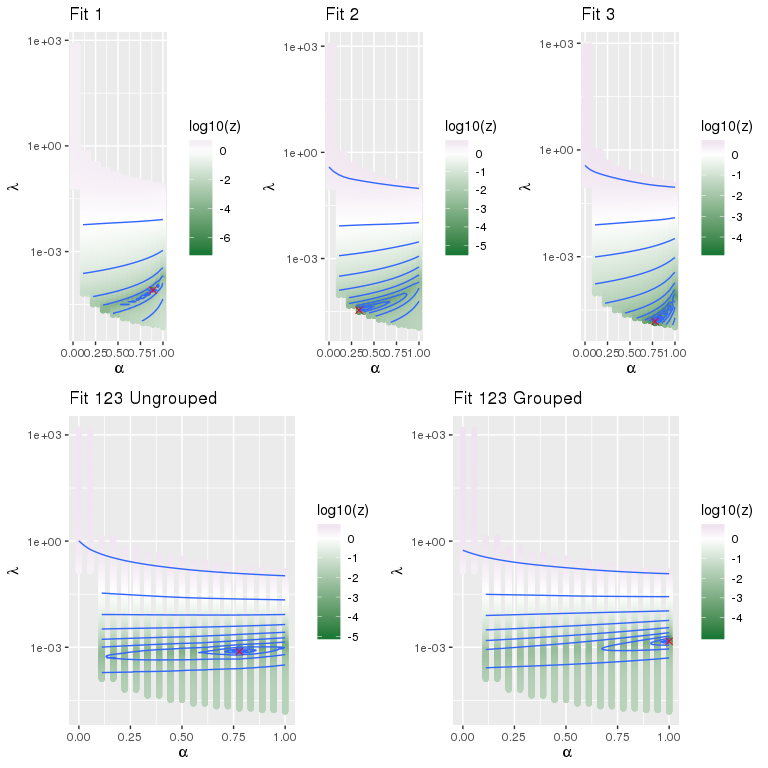The summary model output: all_summaries <- rbindlist(list(summary(fit_1), summary(fit_2), summary(fit_3), summary(fit_123_ungrouped), summary(fit_123_grouped)), idcol = "fit") all_summaries[, fit := factor(fit, 1:5, c("Fit 1", "Fit 2", "Fit 3", "Fit 123 Ungrouped", "Fit 123 Grouped"))] The models with the lowest mean cross validation error are: all_summaries[, .SD[cvm == min(cvm)], by = fit] ## fit l_index lambda cvm nzero alpha ## 1: Fit 1 17 7.967840e-05 0.2337954 13 0.8888889 ## 2: Fit 2 7 3.481007e-05 0.1266873 13 0.3333333 ## 3: Fit 3 15 1.509639e-05 0.1790918 13 0.7777778 ## 4: Fit 123 Ungrouped 15 7.541286e-04 0.4678279 9 0.7777778 ## 5: Fit 123 Grouped 19 1.472763e-03 0.4670949 13 1.0000000 ggplot( all_summaries[, .SD[cvm == min(cvm)], by = c("fit", "nzero")] ) + theme_bw() + aes(x = nzero, y = cvm, color = factor(fit), linetype = factor(fit)) + geom_point() + geom_line()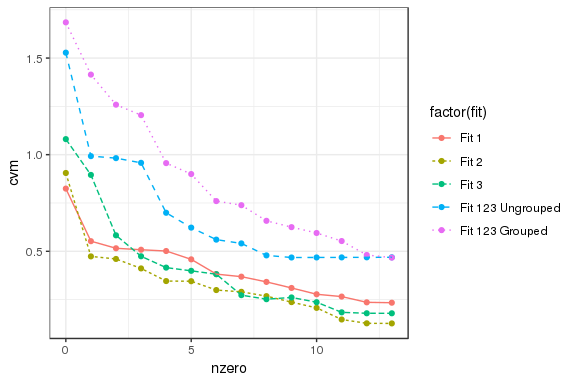It appears that either an ungrouped model with 9 non-zero coefficients or a grouped model with 14 non-zero coefficients would be preferable. Here are the models with the lowest mean cross validation error and eight non-zero coefficients: pref_models <- all_summaries[nzero == 8, .SD[cvm == min(cvm)], by = c("fit")] pref_models ## fit l_index lambda cvm nzero alpha ## 1: Fit 1 19 0.004820748 0.3415076 8 1 ## 2: Fit 2 19 0.005776202 0.2678314 8 1 ## 3: Fit 3 19 0.002850449 0.2520190 8 1 ## 4: Fit 123 Ungrouped 19 0.002696567 0.4787501 8 1 ## 5: Fit 123 Grouped 19 0.017486252 0.6576588 8 1 Let’s look at the coefficients for these models, starting with the three univariate models cbind( coef(fit_1[[pref_models$l_index]], s = pref_models$lambda) , coef(fit_2[[pref_models$l_index]], s = pref_models$lambda) , coef(fit_3[[pref_models$l_index]], s = pref_models$lambda) ) ## 14 x 3 sparse Matrix of class "dgCMatrix" ## 1 1 1 ## (Intercept) -3.2001456 -3.4045561 -3.2863430 ## female . -0.1797073 . ## pcode1 . 1.6923785 -0.7055764 ## pcode2 . 4.8720095 -4.8645557 ## pcode3 0.1507220 . 2.3282238 ## pcode4 . . . ## pcode5 5.1595230 . -1.5361060 ## pcode6 . 0.3386444 . ## ncode1 1.1841171 0.1919097 . ## ncode2 1.9360508 -0.9565740 6.7173951 ## ncode3 0.8290263 -1.9659297 4.9937460 ## ncode4 -2.6370294 3.0401497 -2.0221182 ## ncode5 -2.5132967 . . ## ncode6 0.3277140 . -0.1911358 The following are the non-zero coefficients for the ungrouped models. do.call(cbind, coef(fit_123_ungrouped[[pref_models$l_index]], s = pref_models$lambda)) ## 14 x 3 sparse Matrix of class "dgCMatrix" ## 1 1 1 ## (Intercept) -0.33241922 -0.3313577 0.66377695 ## female 0.24506336 -0.6819977 . ## pcode1 . 1.2978765 -0.55789734 ## pcode2 . 3.2520292 -1.68280619 ## pcode3 . -0.2329660 0.44903238 ## pcode4 0.01753463 . . ## pcode5 3.42782312 -0.2534430 . ## pcode6 . 0.3133426 . ## ncode1 0.72614031 . -0.32975748 ## ncode2 . -2.7979979 0.28323480 ## ncode3 . -2.3084144 0.63521764 ## ncode4 -2.22002122 2.4521624 . ## ncode5 -2.02221356 . 0.08590612 ## ncode6 . . -0.84682767 The grouped results are: do.call(cbind, coef(fit_123_grouped[[pref_models$l_index]], s = pref_models\$lambda))
## 14 x 3 sparse Matrix of class "dgCMatrix"
##                       1           1          1
## (Intercept) -0.20663855 -0.30975585  0.5163944
## female       .           .           .
## pcode1      -0.02412095  0.44687252 -0.4227516
## pcode2      -0.39871732  1.92734453 -1.5286272
## pcode3       .           .           .
## pcode4       .           .           .
## pcode5       1.54552391 -0.73660234 -0.8089216
## pcode6       .           .           .
## ncode1       0.15712998 -0.01067729 -0.1464527
## ncode2       0.22187630 -0.91393942  0.6920631
## ncode3       0.11302591 -0.80565090  0.6926250
## ncode4      -0.98598671  1.10067362 -0.1146869
## ncode5      -0.24127230  0.04007013  0.2012022
## ncode6       .           .           .

ensr can also analyze multivariate Gaussian responses. See the documentation help("glmnet", package = "glmnet") for details.

# 4 Alternative Approaches

Our ensr package is not the only approach to searching for $$\labmda$$ and $$\alpha$$. The glmnetUtils (Microsoft and Ooi 2017) is the most notable comparative package. We encourage the reader to explore the glmnetUtils package and determine if it meets your needs.

There are two major differences in the implementation of ensr and glmnetUtils. The first major difference is that glmnetUtils allows users to specify glmnet::glmnet models with a formula, e.g., y ~ x1 + x2 + x3, whereas ensr maintains the glmnet requirement of the user providing y and x matrices. We opted for the simplicity of staying with glmnet::glmnet arguments for programming efficiency and as a check on reasonable models. It is likely that a model with a factor on the right-hand side of the formula should not be evaluated with elastic net. There does not appear to be a check or warning in glmnetUtils for factor or character (which will be coerced to a factor) variables on the right-hand side of the formula statement. ensr and glmnet, by requiring the user to specify the response and support matrices, forces the user to be aware of and explicitly handle possible character/factor predictor variables. Binary factors are non-trivial in this context as well, but are considerably less difficult to deal with then factors with three or more possible values.

The second major difference between ensr and glmnetUtils is that ensr builds a $$\labmda$$-$$\alpha$$ grid and evaluates glmnet::cv.glmnet at least twice for each value of $$\lambda.$$ For each value of $$\lambda$$, at least two values of $$\alpha$$ will be considered. glmnetUtils only uses the default $$\labmda$$ values for each specific $$\alpha$$ value. By constructing a $$\labmda$$-$$\alpha$$ grid, ensr provides minimally sufficient support for estimating a contour plot for a (x = $$\alpha,$$ y = $$\lambda,$$ z = cross-validation mean error) surface. The glmnetUtils results require imputation before such a surface could be estimated. We feel that ensr’s use of a $$\labmda$$-$$\alpha$$ grid is a significant advantage relative to glmnetUtils.

See the example script compare-to-glmnetUtils.R in the examples directory on the ensr github page: https://github.com/dewittpe/ensr.

# 5 Session Info

print(sessionInfo(), local = FALSE)
## R Under development (unstable) (2019-01-13 r75986)
## Platform: x86_64-pc-linux-gnu (64-bit)
## Running under: Debian GNU/Linux 9 (stretch)
##
## Matrix products: default
## BLAS/LAPACK: /usr/lib/libopenblasp-r0.2.19.so
##
## attached base packages:
##  parallel  stats     graphics  grDevices utils     datasets  methods
##  base
##
## other attached packages:
##   doMC_1.3.5        iterators_1.0.10  ggforce_0.1.3
##   ggplot2_3.1.0     data.table_1.12.0 ensr_0.1.0
##   glmnet_2.0-16     foreach_1.4.4     Matrix_1.2-15
##  qwraps2_0.4.0     knitr_1.21
##
## loaded via a namespace (and not attached):
##   Rcpp_1.0.0       highr_0.7        bindr_0.1.1      compiler_3.6.0
##   pillar_1.3.1     plyr_1.8.4       tools_3.6.0      digest_0.6.18
##   evaluate_0.12    tibble_2.0.1     gtable_0.2.0     lattice_0.20-38
##  pkgconfig_2.0.2  rlang_0.3.1      yaml_2.2.0       xfun_0.4
##  bindrcpp_0.2.2   gridExtra_2.3    withr_2.1.2      stringr_1.3.1
##  dplyr_0.7.8      tidyselect_0.2.5 grid_3.6.0       glue_1.3.0
##  R6_2.3.0         rmarkdown_1.11   farver_1.1.0     tweenr_1.0.1
##  purrr_0.2.5      magrittr_1.5     units_0.6-2      MASS_7.3-51.1
##  scales_1.0.0     codetools_0.2-16 htmltools_0.3.6  assertthat_0.2.0
##  colorspace_1.4-0 labeling_0.3     stringi_1.2.4    lazyeval_0.2.1
##  munsell_0.5.0    crayon_1.3.4